Question

# Design and implement a MEALY finite state machine that would detect a sequence 0110 in the...

Design and implement a MEALY finite state machine that would detect a sequence 0110 in the input stream. Overlapping sequences are allowed.

A) draw state diagram You would need no more than 4 states to implement the logic

B) tabulate the state transition table

C) show the implementation of the FSM using D-flip-flops

We need at least 10 more requests to produce the answer.

0 / 10 have requested this problem solution

The more requests, the faster the answer.

All students who have requested the answer will be notified once they are available.

#### Earn Coins

Coins can be redeemed for fabulous gifts.

Similar Homework Help Questions
• ### Question 6. (20 points) You are designing a sequence detector that can detect 0110', if this sequ...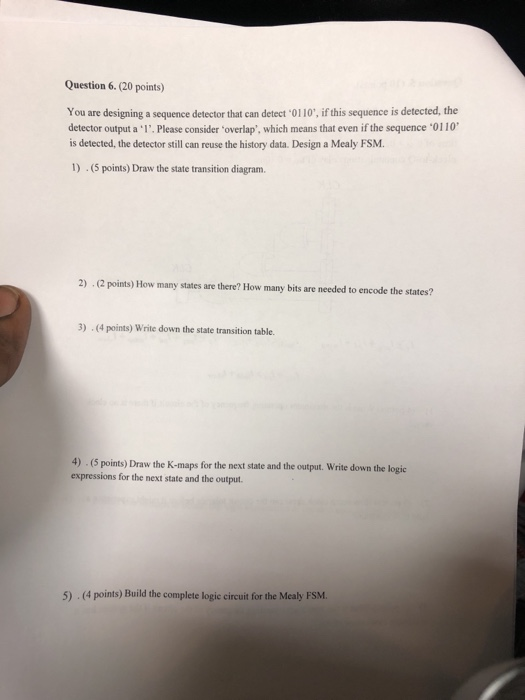Question 6. (20 points) You are designing a sequence detector that can detect 0110', if this sequence is detected, the detector output a 1'. Please consider 'overlap', which means that even if the sequence '0110 is detected, the detector still can reuse the history data. Design a Mealy FSM. 1) .(5 points) Draw the state transition diagram. 2) (2 points) How many states are there? How many bits are needed to encode the states? 3) -(4 points) Write down the...

• ### Design a 4-bit serial bit sequence detector. The input to your state detector is called DIN...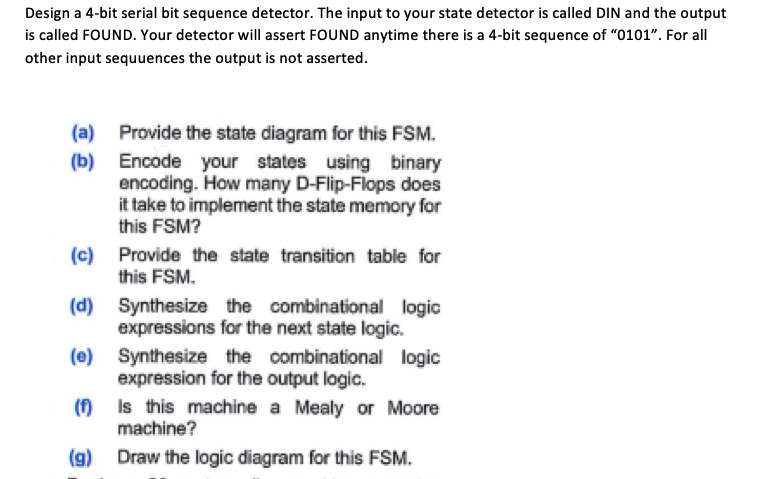Design a 4-bit serial bit sequence detector. The input to your state detector is called DIN and the output is called FOUND. Your detector will assert FOUND anytime there is a 4-bit sequence of "0101". For all other input sequuences the output is not asserted. (a) (b) Provide the state diagram for this FSM. Encode your states using binary encoding. How many D-Flip-Flops does it take to implement the state memory for this FSM? (c) Provide the state transition table...

• ### Answer both parts please 3. Implement a Mealy FSM to detect the "1100110” sequence with overlap....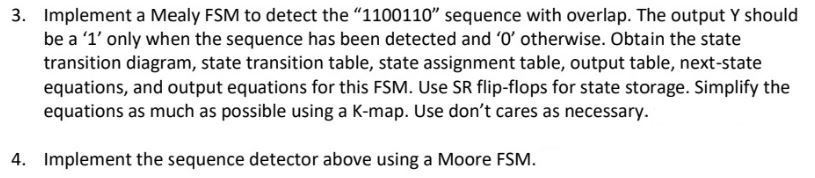Answer both parts please 3. Implement a Mealy FSM to detect the "1100110” sequence with overlap. The output Y should be a 'l' only when the sequence has been detected and 'O' otherwise. Obtain the state transition diagram, state transition table, state assignment table, output table, next-state equations, and output equations for this FSM. Use SR flip-flops for state storage. Simplify the equations as much as possible using a K-map. Use don't cares as necessary. 4. Implement the sequence detector...

• ### Design a sequential circuit for a sequence detector that detects the sequence 10011

Design a sequential circuit for a sequence detector that detects the sequence 10011. A continuous bit stream is fed at the input of the circuit. Every time the circuit detects the sequence 10011, an output line is made HIGH a) Sketch Mealy FSM for the sequence detector. b) Tabulate the state table c) Using K-maps, write down the simplified Boolean expressions of the flip-flops input equations using T flip-flops d) Sketch the logic circuit diagram

• ### 4) Design FSMs that will detect the following sequence (including overlapping sequences). When the sequence is...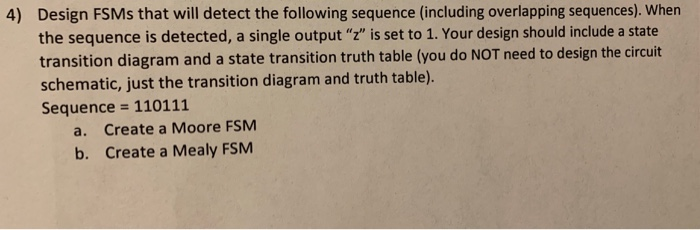4) Design FSMs that will detect the following sequence (including overlapping sequences). When the sequence is detected, a single output "z" is set to 1. Your design should include a state transition diagram and a state transition truth table (you do NOT need to design the circuit schematic, just the transition diagram and truth table). Sequence = 110111 a. Create a Moore FSM b. Create a Mealy FSM

• ### Design a sequential circuit for a single-input and single output Moore-type FSM that produces an ...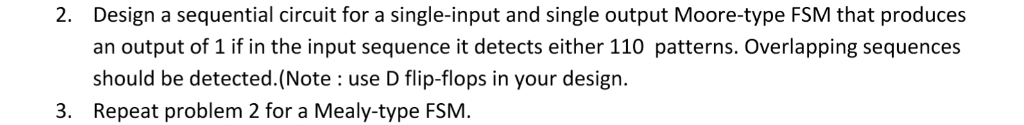digital logic Design a sequential circuit for a single-input and single output Moore-type FSM that produces an output of 1 if in the input sequence it detects either 110 patterns. Overlapping sequences should be detected.(Note : use D flip-flops in your design. Repeat problem 2 for a Mealy-type FSM 2. 3. Design a sequential circuit for a single-input and single output Moore-type FSM that produces an output of 1 if in the input sequence it detects either 110 patterns. Overlapping...

• ### Design a MOORE FINITE STATE MACHINE for a Sequence Detector that detects sequentially the number 1510 in a stream of input bits

Design a MOORE FINITE STATE MACHINE for a Sequence Detector that detects sequentially the number 1510 in a stream of input bits. Label the input w. The output z is equal to 1 if the number 1510 was detected. After detecting the pattern (1510), the machine goes back in the initial state S0. a) Draw the state diagram for the FSM. Add an asynchronous Reset, active LOW. b) How many FFs do you need to implement this FSM? Note: Label the states S0,...

• ### Problem 02 Design a finite-state machine that records the results of flipping a coin a certain nu...Problem 02 Design a finite-state machine that records the results of flipping a coin a certain number of times. The state machine takes as an input flip which is zero for heads and one for tails. The states are labeled so as to indicate the numbers of heads and the nurnber of tails, NONE. H. T, HH, TT HT, HHH, etc. The order that the heads or tails was flipped does not matter, only the total numbers of heads and...

• ### Design and Draw the Circuit Schematic for the FSM if it were a Mealy Machine. Your...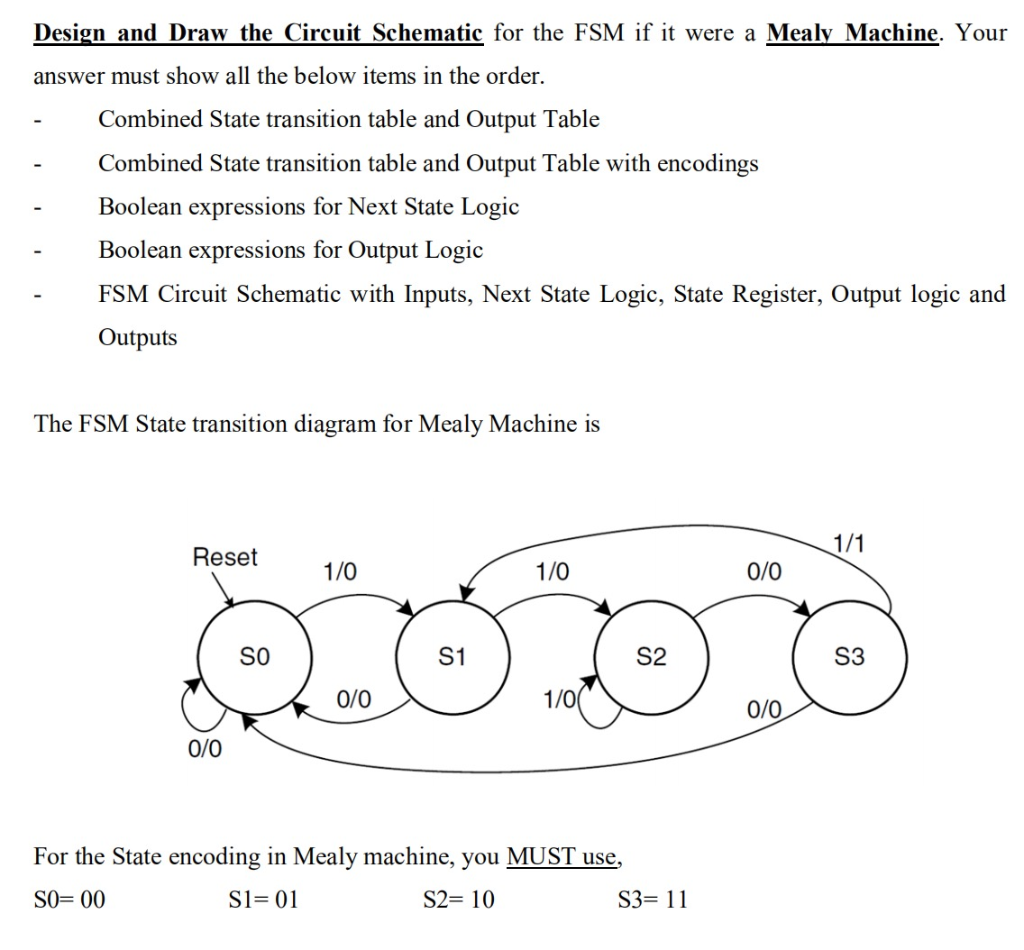Design and Draw the Circuit Schematic for the FSM if it were a Mealy Machine. Your answer must show all the below items in the order. Combined State transition table and Output Table Combined State transition table and Output Table with encodings Boolean expressions for Next State Logic Boolean expressions for Output Logic FSM Circuit Schematic with Inputs, Next State Logic, State Register, Output logic and Outputs The FSM State transition diagram for Mealy Machine is 1/1 Reset 1/0 1/0...

• ### finite State Machine

Design finite state machine to detect if there is sequence 101 ininput series x . If 101 found, set an output flag Y=1 , otherwiseY=0.1)numer of State is =?2)number of State variable =?3)Drive the next state transition table and outputtable4)SOP Logic expression for next state.5)SOP logic expression for output.6)Draw the circuit diagram.<<<<<<<<PLEASE ANSWER ALL THE QUESTION>>>>>>>>>>>